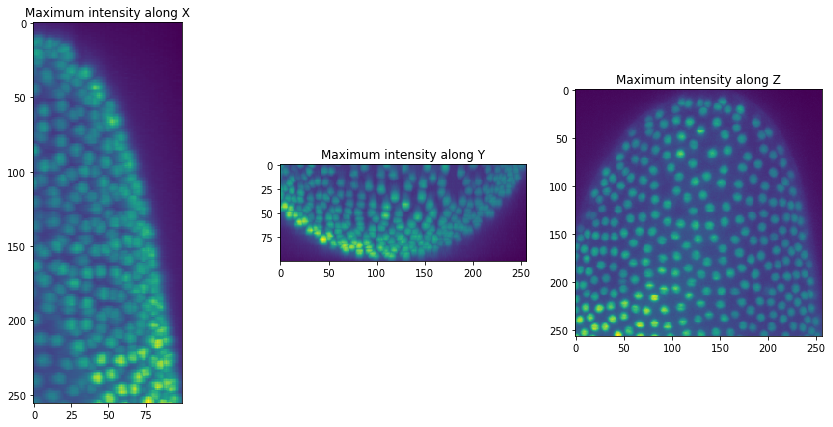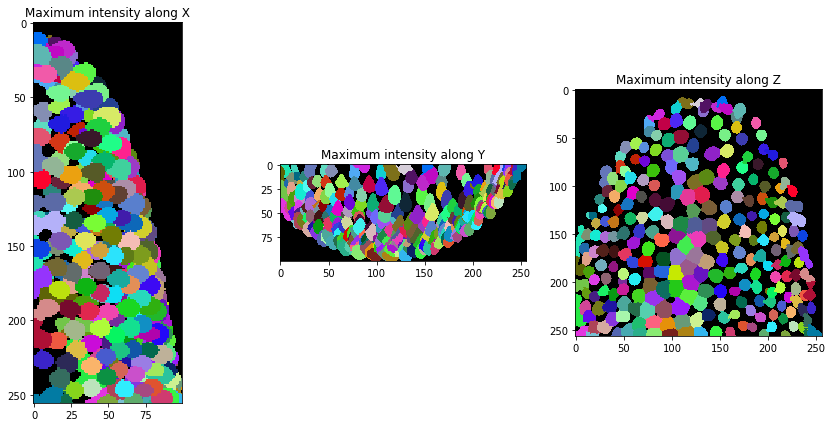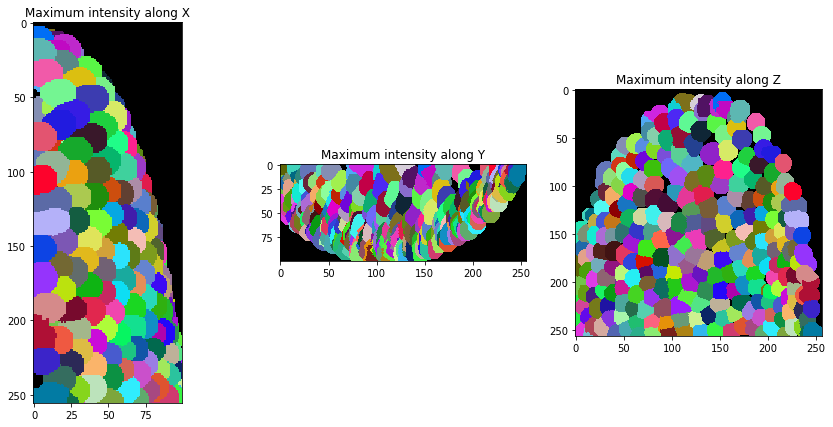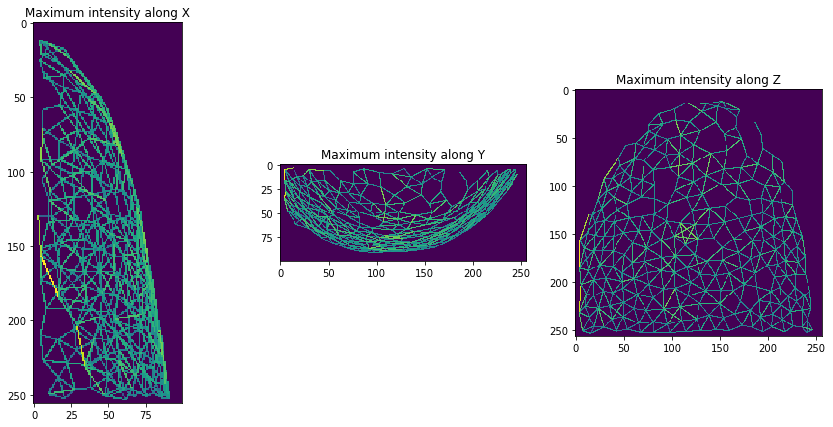# Neighbor meshes in three dimensions#

The neighbor meshes we worked with in this chapter in general also work in three dimensions. It is just more challenging to visualize those.

from skimage.io import imread
import pyclesperanto_prototype as cle
import matplotlib.pyplot as plt


The image data we use here shows a crop of a developing Tribolium castaneum embryo imaged using light sheet microscopy by Daniela Vorkel, Myers lab, MPI-CBG / CSBD Dresden.

raw_image = imread("../../data/Lund_000500_resampled-cropped.tif")

raw_image.shape

(100, 256, 256)


For the ease-of-use we write a short function for visualizing our image stack in three maximum projections from different persepctives.

def orthogonal_show(image, labels=False):

fig, axs = plt.subplots(1, 3, figsize=(15, 7))

cle.imshow(cle.maximum_x_projection(image), plot=axs, labels=labels)
cle.imshow(cle.maximum_y_projection(image), plot=axs, labels=labels)
cle.imshow(cle.maximum_z_projection(image), plot=axs, labels=labels)

axs.set_title("Maximum intensity along X")
axs.set_title("Maximum intensity along Y")
axs.set_title("Maximum intensity along Z")

orthogonal_show(raw_image)We can now segment nuclei in our our dataset.

background_subtracted = cle.top_hat_box(raw_image, radius_x=5, radius_y=5, radius_z=5)

nuclei = cle.voronoi_otsu_labeling(background_subtracted)

orthogonal_show(nuclei, labels=True)After segmentation, we expand the labels a bit so they touch.

expanded_nuclei = cle.dilate_labels(nuclei, radius=4)

orthogonal_show(expanded_nuclei, labels=True)And then, we can visualize a (centroid) distance mesh between touching neighbors.

mesh = cle.draw_distance_mesh_between_touching_labels(expanded_nuclei)

orthogonal_show(mesh)